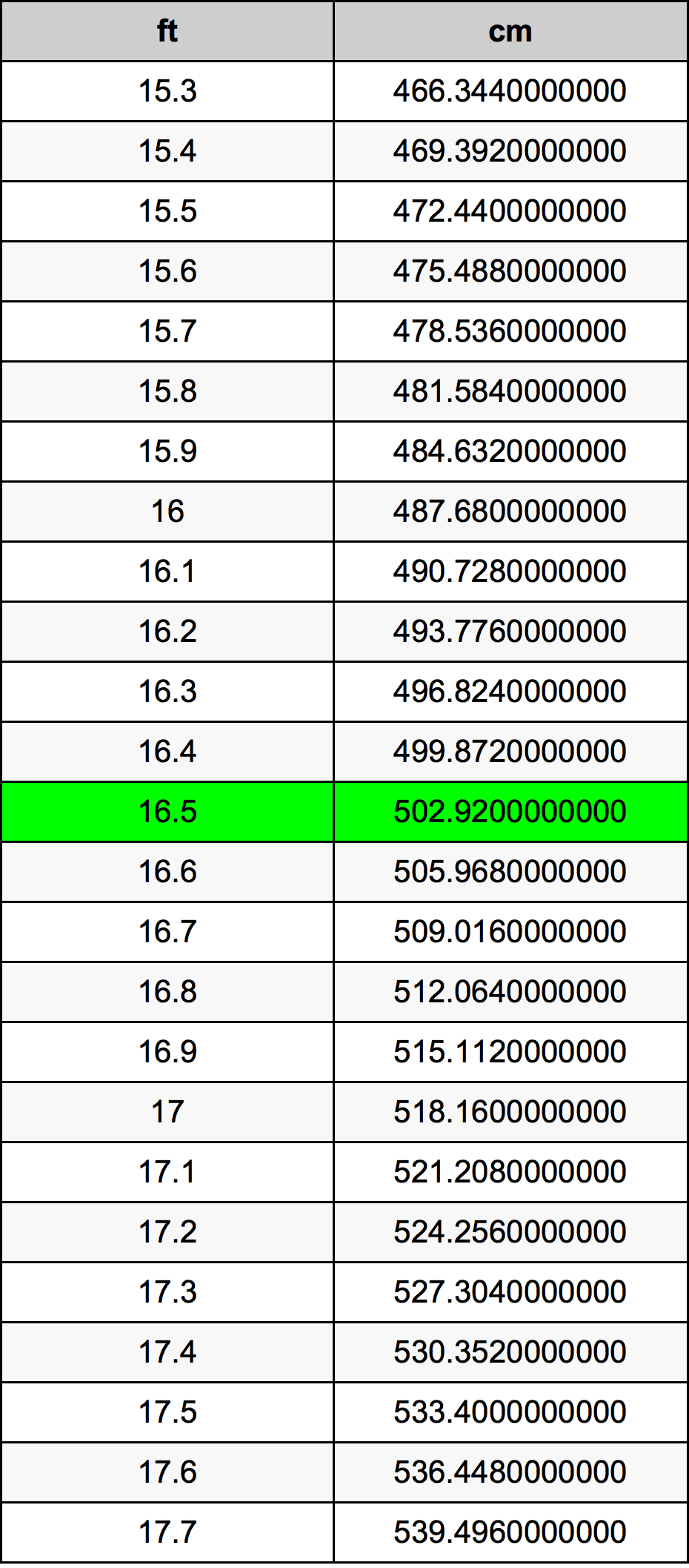Feet To Cm

# 16.5 ft to cm16.5 Feet to Centimeters

ft
=
cm

## How to convert 16.5 feet to centimeters?

 16.5 ft * 30.48 cm = 502.92 cm 1 ft
A common question is How many foot in 16.5 centimeter? And the answer is 0.5413385827 ft in 16.5 cm. Likewise the question how many centimeter in 16.5 foot has the answer of 502.92 cm in 16.5 ft.

## How much are 16.5 feet in centimeters?

16.5 feet equal 502.92 centimeters (16.5ft = 502.92cm). Converting 16.5 ft to cm is easy. Simply use our calculator above, or apply the formula to change the length 16.5 ft to cm.

## Convert 16.5 ft to common lengths

UnitLength
Nanometer5029200000.0 nm
Micrometer5029200.0 µm
Millimeter5029.2 mm
Centimeter502.92 cm
Inch198.0 in
Foot16.5 ft
Yard5.5 yd
Meter5.0292 m
Kilometer0.0050292 km
Mile0.003125 mi
Nautical mile0.0027155508 nmi

## What is 16.5 feet in cm?

To convert 16.5 ft to cm multiply the length in feet by 30.48. The 16.5 ft in cm formula is [cm] = 16.5 * 30.48. Thus, for 16.5 feet in centimeter we get 502.92 cm.

## 16.5 Foot Conversion Table## Alternative spelling

16.5 ft to Centimeters, 16.5 ft in Centimeters, 16.5 Foot to Centimeters, 16.5 Foot in Centimeters, 16.5 ft to cm, 16.5 ft in cm, 16.5 ft to Centimeter, 16.5 ft in Centimeter, 16.5 Feet to Centimeter, 16.5 Feet in Centimeter, 16.5 Feet to cm, 16.5 Feet in cm, 16.5 Feet to Centimeters, 16.5 Feet in Centimeters